# Capacitance Meter

A capacitance meter is a piece of electronic test equipment whose purpose is to measure capacitance, mostly of discrete capacitors. The capacitance meter works based off of the directly proportional relationship between capacitance and a time constant.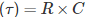This relation is used in this method of measurement. So, we can first go through a simple RC circuit with a supply voltage of VIN (shown below).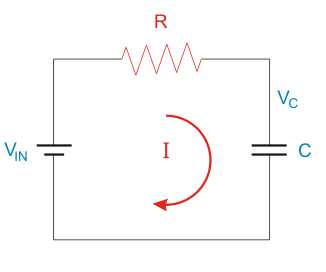During the charging period of the capacitor, the voltage across the capacitor at any instant is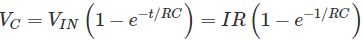The time needed to charge the capacitor to exactly 63.5 percent of the total input voltage.
is termed as time constant. It is denoted by ‘τ’.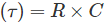Now, Assume a capacitor which is charged using a constant current source and the capacitor gets discharged through a resistor having a fixed resistance. To measure the capacitance of this circuit, we can implement a 555 timer together with a few digital test apparatus. The clear way of measuring the capacitance is by measuring the time period of oscillations. The reading can be obtained directly in nanofarads or microfarads by selecting the exact size of the charging resistance.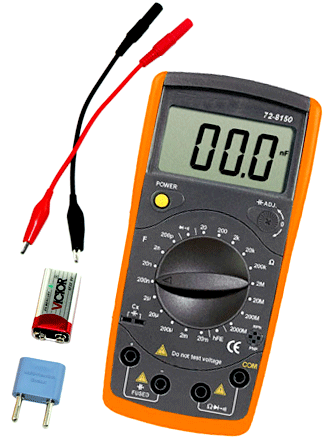When compared to other capacitance measuring techniques, this meter is capable to hold electrolytic which is up to tens of thousands of Farad.

If the testing capacitor has any leakage, this method will create the value of the capacitance to be smaller than the actual value. This method is also an efficient indicator of the behavior of the test capacitor in the majority bypass and timing circuits. The block diagram of a basic digital capacitance meter with the 555 timer IC is shown below.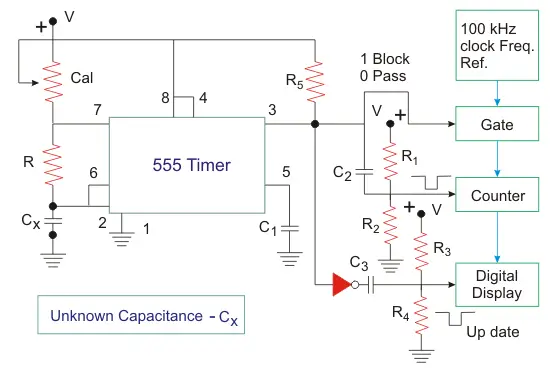Here, we can see a 555 timer in the circuit. It works as an astable multivibrator. The frequency of this multivibrator is governed by the unknown capacitance value (CX). The output of this multivibrator is connected to a digital counter. This counter can measure the length of the cycle of the square wave.
Cycle length of the square wave which is formed by 555 timers can be calculated using the formula: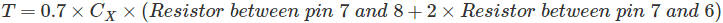On the peak value of the charging curve, a digital counter will get reset. At this time, a clock of 100 kHz pulses is made ON and it is routed to the counter. Next, after the completion of discharge part of the cycle, the display will get updated and we can simply read out the value of the capacitor. To get the direct display of the capacitance value simply and correctly, the selection of charging currents and reference frequency should be apt.

The shielding of leads should be made assure and for low capacity measurements, it should be kept short. This is because the hum of 50 Hz can result in some minor instability.

Want To Learn Faster? 🎓
Get electrical articles delivered to your inbox every week.
No credit card required—it’s 100% free.